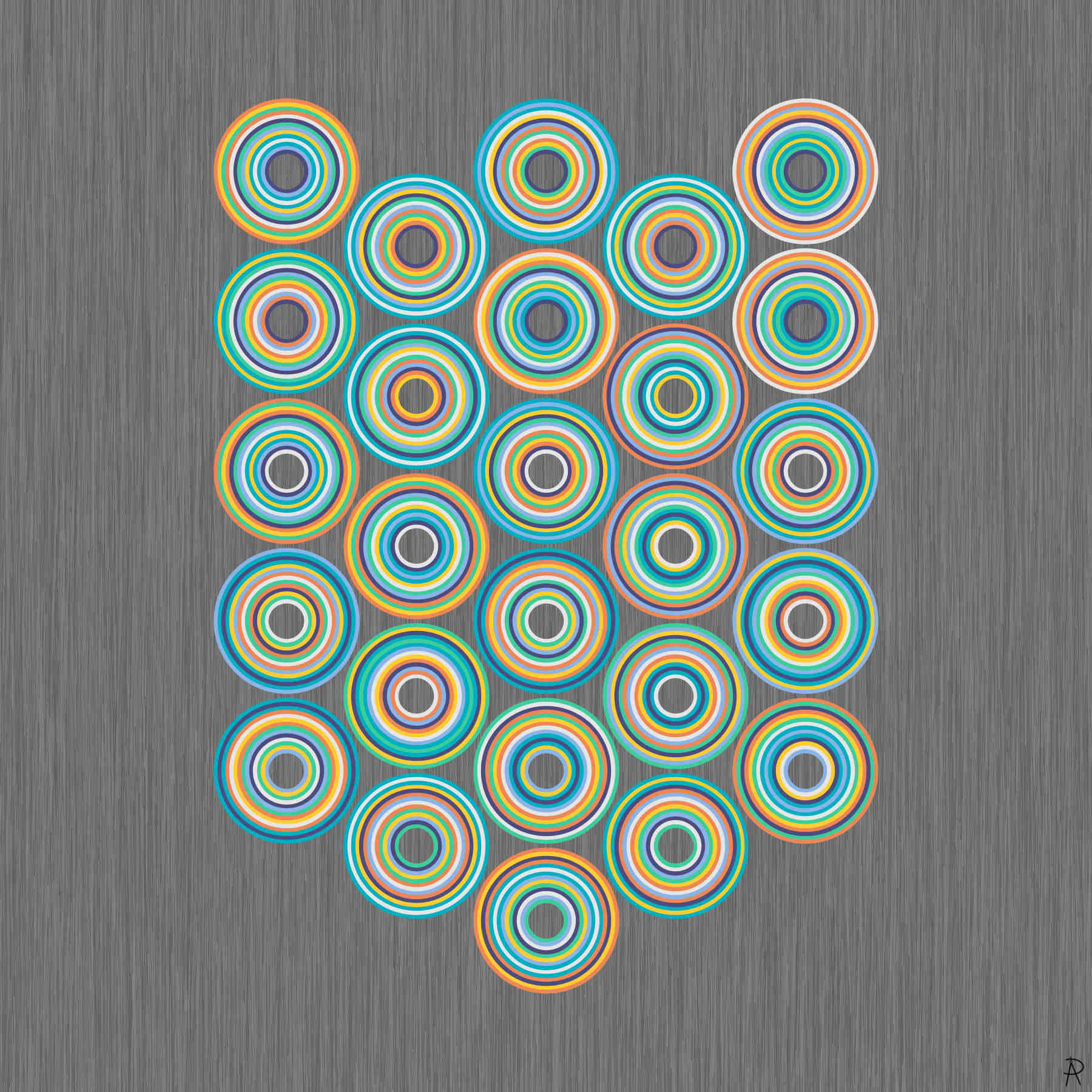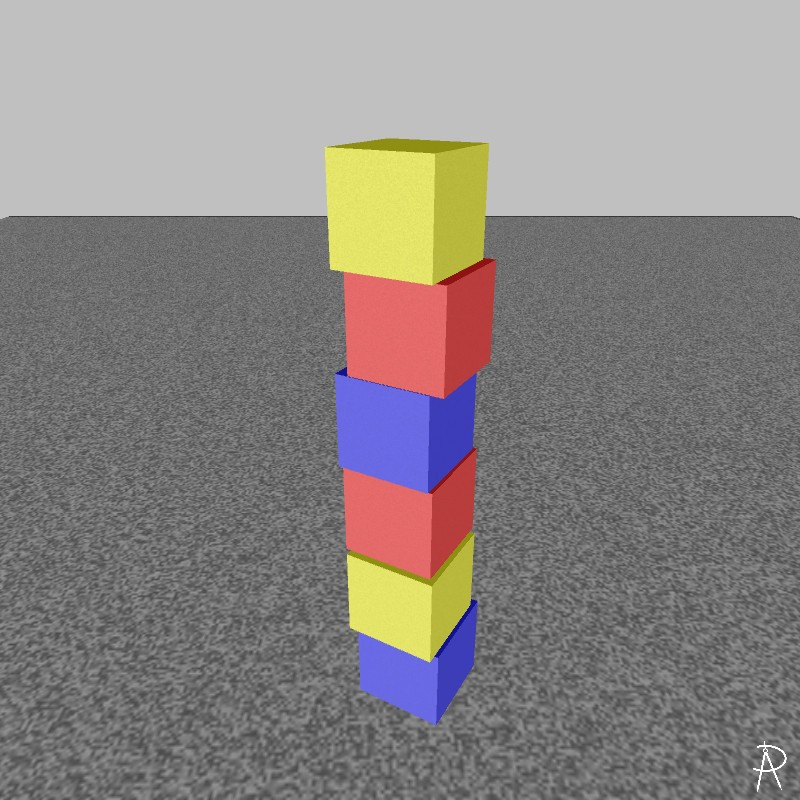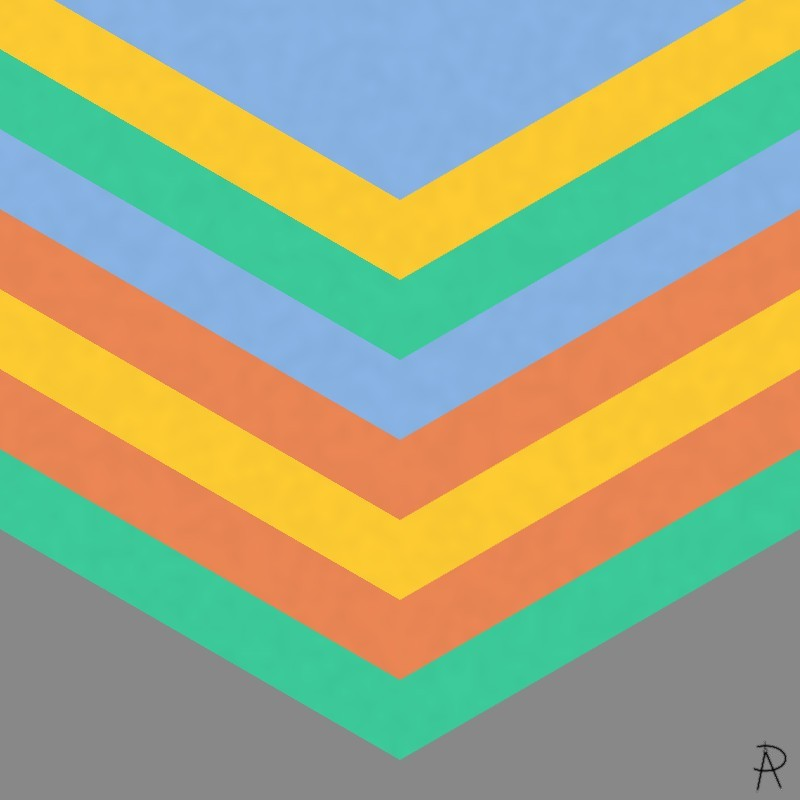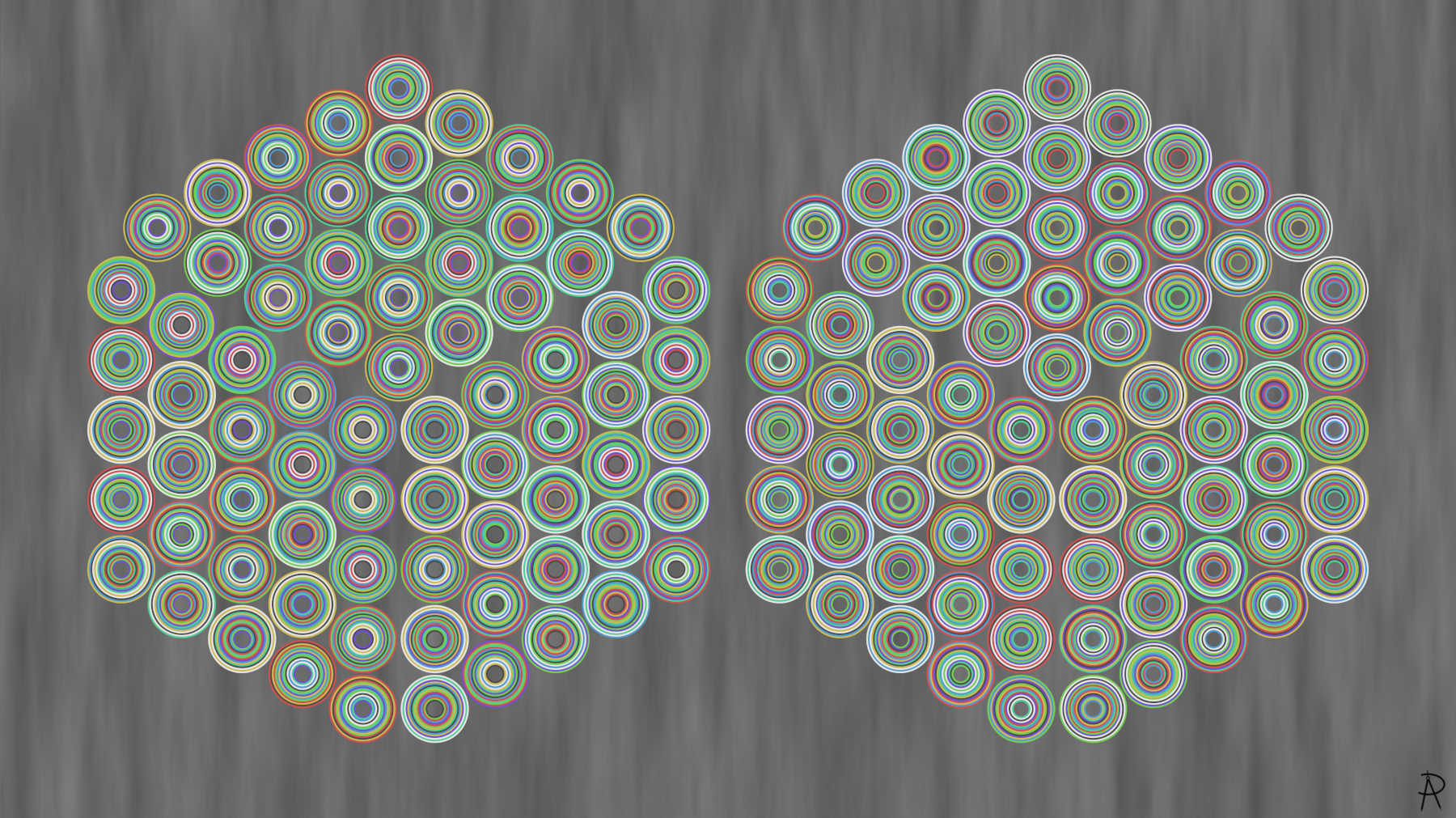Septenary Circles Digital Print 2021

This artwork answers the question of how many ways can one place seven color pairs such that there is one other color between the first color pair, two colors between the second color pair, and so on. Mathematically, these are the twenty-six different Langford sequences for $$n = 7$$.

### Award

I was honored to have this piece win The American Mathematical Society's Best photograph, painting, or print JMM Mathematical Art Exhibition Award, given for aesthetically pleasing works that combine mathematics and art. The award was established in 2008 through an endowment provided to the American Mathematical Society by an anonymous donor who wishes to acknowledge those whose works demonstrate the beauty and elegance of mathematics expressed in a visual art form. See the works in the 2022 Joint Mathematics Meetings Gallery.

### Background and Inspiration

A Langford sequence, named for C. Dudley Langford (October 16, 1905 – January 11, 1969), is a permutation of the integers $$1, 1, 2, 2, 3, 3, \ldots, n, n$$ for some positive integer $$n$$ where the gap between the first and second occurrence of a value $$k$$ is $$k$$. These sequences are typically written as a juxtaposition of the digits for small values of $$n$$. There is one Langford sequence of order 3 (312132) and one of order four (41312432). A Langford sequence and its reverse are considered equivalent.

Langford's account of the origin of these sequences was published in 1958:

Years ago, my son, then a little boy, was playing with some coloured blocks. There were two of each colour, and one day I noticed that he had placed them in a single pile so that between the red pair there was one block, two between the blue pair, and three between the yellow. I then found that by a complete rearrangement I could add a green pair with four between them.

Langford went on to provide several examples of these sequences, with colors replaced with integers representing the number of colors between two identical colors. He invited others to provide a theoretical understanding of these sequences.

In 1959, Davies proved a Langford sequence exists when its length is $$4m$$ or $$4m-1$$ for positive integer $$m$$. Thus, there are no Langford sequences of lengths 1, 2, 5, 6, 9, 10, etc.

There are 26 such permutations when $$n = 7$$:
74151643752362, 73625324765141, 73161345726425, 72632453764151, 72462354736151, 72452634753161, 71416354732652, 71316435724625, 62742356437151, 61517346532472, 57416154372632, 57263254376141, 57236253471614, 57141653472362, 56171354632742, 53672352461714, 53647352462171, 52732653417164, 52472654131763, 51716254237643, 46357432652171, 46171452632753, 45671415362732, 37463254276151, 35743625427161, 34573641512762.
These are the sequences depicted in this artwork. Seven colors are used and a specific color represents the same gap size in each annulus.

The number of sequences of a given length rises rapidly. According to the OEIS, there are 150 sequences when $$n=8$$ and 17792 sequences when $$n=11$$.

Martin Gardner's Mathematical Games column from the November 1967 Scientific American gave new attention on these sequences. Computer searches yielded new results on the number of sequences for a given $$n$$. Gardner wrote

There are no solutions to Langford's problem, as it is now called with five or six pairs of cards. There are 25 distinct solutions with seven pairs. No one knows how to determine the number of distinct solutions for a given number of pairs except by exhaustive trial-and-error methods, but perhaps the reader can discover a simple method of determining if there is a solution.

Gardner revisited this problem for one of the reprintings of collections of his Mathematical Games articles. He noted that in 1971 it was determined that there are actually 26 solutions when $$n=7$$ and 150 when $$n=8$$. Furthermore, there are 17,792 solutions when $$n=11$$ and 108,144 solutions when $$n=12$$, independently confirmed by E.J. Groth and John Miller.

Langford sequences are related to Skolem sequences. I became aware of these sequences at a Math/CS colloquium talk given by Heather Jordon at Albion College in 2012. Artistically, I was inspired by Bridget Riley's stripe paintings, such as Cool Edge (1982), through a talk by Neil Dodgson at the Bridges Enschede conference (2013).

### Related WorksLangford's Blocks Digital Print, 2021 This work depicts the origins of Langford sequences as described above and illustrates the unique Langford sequence of order 3. The sequence 312132 is a representation of the set of block colorings, with 1 representing red blocks, 2 representing blue blocks, and 3 representing yellow blocks.Chevronels Digital Print, 2021 This work depicts the origins of Langford sequences as described above and illustrates the unique Langford sequence of order 4. The sequence 23421314 is a representation of the set of block colorings, with 1 representing red blocks, 2 representing blue blocks, 3 representing yellow blocks, and 4 representing green blocks.Archipelago of Octadics Digital Print, 2021 This artwork answers the question of how many ways can one place eight color pairs such that there is one other color between the first color pair, two colors between the second color pair, and so on. Mathematically, these are the 150 different Langford sequences for $$n=8$$. This piece is the fourth in a series of artworks related to Langford sequences.

### Epilogue

Langford died January 11, 1969 at the age of 63. The The Mathematical Gazette published Langford's obituary. It stated,

Dr. Langford was well known to members of the Mathematical Association as a staunch and ingenious contributor to The Gazette, but not many knew the serious disabilities against which he struggled for many years with unfailing courage. The effects of polio, which had attacked him at the age of 12, now began to be felt and a series of fractures, caused by falls due to muscular weakness, forced him to retire. Pain continued to increase, but his courage and sense of humour never failed him. His wife describes how he made life more pleasant for all who came in contact with him, and a mutual friend, not given to exaggeration, has marvelled at the support that she, on her side, continued to give.

### Publication History

• 2022 Joint Mathematical Meetings Exhibition of Mathematical Art, Edited by Dan Bach, Robert Fathauer, and Nathan Selikoff, 2021.

### References

• C. Dudley Langford. Problem. The Mathematical Gazette, vol. 42, no. 341, 1958, pp. 228–228. JSTOR, www.jstor.org/stable/3610395.
• In November 1967, Martin Gardner, Mathematical Games: A mixed bag of logical and illogical problems to solve, Scientific American, November 1967.
• Roy O. Davies. On Langford's Problem (II). The Mathematical Gazette, vol. 43, no. 346, 1959, pp. 250–255. JSTOR, www.jstor.org/stable/3610650.
• Neil Dodgson, Mathematical characterization of Bridget Riley's stripe paintings, Journal of Mathematics and the Arts, Volume 6, Issue 2--3, 2012.
• Sequence A014552 in the On-Line Encyclopedia of Integer Sequences (OEIS.org).
• E.A.M., Obituary: C. Dudley Langford, The Mathematical Gazette, vol. 53, no. 385, December 1969, 314.